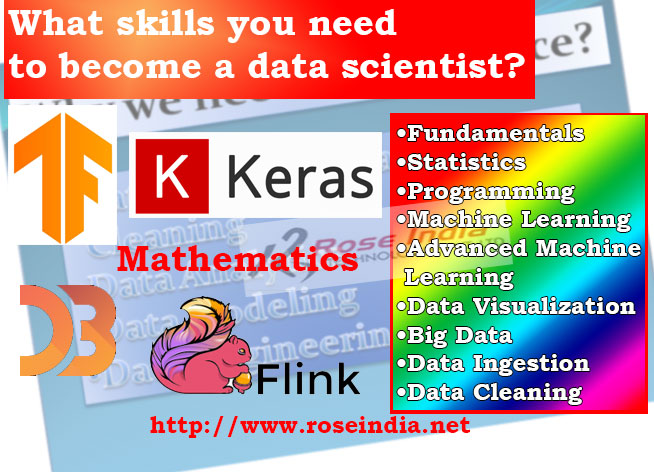# What skills you need to become a data scientist?

In this article we are discussing about the data science and explain you what skills you need to become a data scientist?

In this article we are discussing about the data science and explain you what skills you need to become a data scientist?

## Learning Data Science: What skills you need to become a data scientist?

We are going to discuss about the skills that you must master to become a productive and successful data scientist. The main job role of a data scientist includes collecting, processing and analyzing large amount of data using various branch of science. Data Scientist spends over 70% of time in data preparation activities such as data collection, data clearing, data filtering and data analysis. Once data is cleaned are ready for machine learning, then data scientists train and use various machine learning models to analyze the data.

In this section we are going to explore the technologies one should learn to become productive data scientists. Data Scientists must learning programming, mathematics, machine learning frameworks, machine learning algorithms and data visualization tools. So, there are lot of things that you must learn to become a skilled data scientists.

If you are aspiring to be a data scientist, it is important to acquire certain skills besides having an in-depth knowledge of the field. A data scientist must have the following skills.• Fundamentals
• Statistics
• Programming
• Machine Learning and Advanced Machine Learning (Deep Learning)
• Data Visualization
• Big Data
• Data Ingestion
• Data Cleaning

Let's explain each of these required skills one by one.

### Fundamentals

You need to have solid command on the fundamentals of data science that includes the following.

• Matrices and Linear Algebra Functions
• Hash Functions and Binary Tree
• Relational Algebra, Database Basics
• ETL ( Extract Transform Load )
• Reporting

### Statistics

You need to have a solid command on various fields of statistics that include the following.

• Descriptive Statistics (Mean, Median, Range, Standard Deviation, Variance)
• Exploratory Data Analysis
• Percentiles and Outliers
• Probability Theory
• Bayes Theorem
• Random Variables
• Cumulative Distribution function (CDF)
• Skewness
• Various Statistical Models

## Programming

A data scientist need command over any one programming language. For data science tasks 'R' or 'Python' is considered as most suitable language.

### Machine Learning and Advanced Machine Learning

The data scientist must know how Machine learning works and how it can be utilised for data modelling and algorithms. Some of the key Machine Learning techniques that a data scientist should have knowledge about include the following.

• Supervised Learning
• Unsupervised Learning
• Reinforcement Learning

### Machine Learning Algorithm

Some key Supervised and Unsupervised learning algorithms that you need to know include the following.

• Linear Regression
• Logistic Regression
• Decision Tree
• Random Forest
• K Nearest Neighbour
• Clustering

### Machine learning frameworks and libraries

There are many machine learning programming languages and frameworks for creating machine learning models. These frameworks and libraries provides pre-coded models that can be used to developed your own model. You can these to develop your model from scratch or use existing model and train it with your data to meet your business needs. Here are the list of top machine learning frameworks and libraries used these days:

• TensorFlow
• Microsoft CNTK
• Theano
• Cafee
• Keras
• Torch
• Accord.NET
• Spark MLlib
• Sci-kit Learn
• MLPack
• Amazon Machine Learning
• Microsoft Cognitive Toolkit

Among all these currently TensorFlow, Keras, Sci-kit Learn and Spark MLlib are most popular machine learning libraries. You can define our path and then learn at least 3-4 machine learning frameworks.

### Data Visualization

Data visualization as an important component of the data lifecycle is also a key area of expertise for the aspiring data scientist. Some of the key data visualization tools that a data scientist should be versed with include the following.

• Tableau
• Kibana
• Datawrapper

### Big Data Analytics

Big Data Analytics is a crucial part of data science that helps extracting the hidden insights of the large pull of structured and unstructured data across various niches and categories. Besides having a solid idea about how Big Data analytics work, you need to have a sound knowledge about important frameworks responsible for processing Big Data such as Hadoop and Spark.

### Data Ingestion

Data ingestion is the process responsible for data import, transfer, storage and processing for future. This involves storage solutions and data loading from multiple sources. Some of the key ingestion tools that you need to know include the following.

• Apache Flume
• Apache Sqoop